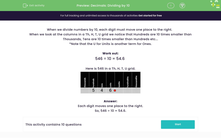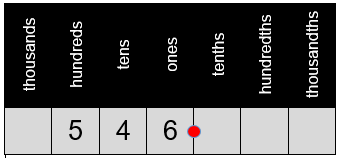# Decimals: Dividing by 10

In this worksheet, students divide numbers by 10 by shifting digits one place to the right in a grid.Key stage:  KS 2

Curriculum topic:   Number: Multiplication and Division

Curriculum subtopic:   Multiply/Divide Using Decimals

Difficulty level:#### Worksheet Overview

When we divide numbers by 10, each digit must move one place to the right.

When we look at the columns in a Th, H, T, O grid we notice that Hundreds are 10 times smaller than Thousands, Tens are 10 times smaller than Hundreds etc...

Work out:

546 ÷ 10 = 54.6

Here is 546 in a Th, H, T, O grid.